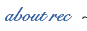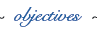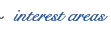In the present era of modern technology, engineering computing plays a fundamental role among all other engineering activities. Engineering computing comprises two main steps, simulation/analysis and optimization/design. The reliability of these two steps depends on how effectively we predict the system behavior and how successfully we achieve optimal solutions not limited to local optima but extend beyond that to obtain global optima.

Reliable Engineering Computing (REC) requires that computing systems accommodate several sources of errors. Looking at a system model as an example, uncertainty can be introduced in four ways:

1.  The appropriateness of the mathematical model to describe the physical system;

2.  The discretization of mathematical model into a computational framework;

3.  The inexact knowledge of input parameters of a problem;

4.  Errors introduced by the nature of computer finite arithmetic.

The center’s objectives are the development of methods and tools for reliable engineering computing, the promotion of reliable computing in the different fields of engineering, and fostering interdisciplinary collaborations between engineering disciplines, computer science and mathematics on one hand, and with industry on the other hand. In addition, the center will focus on promoting the international effort in this endeavor and will seek to host activities in this aspect.

The focus of the REC is based on, but not limited to, interval analysis. Key to the endeavor is the inclusion of uncertainties in engineering systems via the concept of computing on interval quantities (or other sets) instead of computing with real numbers. Such intervals can be interpreted as sets of bounded probability density functions (PDF) and account for model errors and provide robust bounds on model predictions. For example, floating-point computing provides a single number that contains no accuracy information, and most real numbers cannot be represented exactly using finite precision floating-point numbers. Consequently, using single floating-point computations might not be the right way to provide robust engineering solutions. Reliance on large-scale computations may hide issues of accuracy and sensitivity on input data; however, multiple analyses to study the accuracy and sensitivity of a solution may still be computationally prohibitive.

The Center’s interest areas include solid and structural mechanics, geotechnology, heat transfer, fluid mechanics, electrical and magnetic fields, petroleum mining, biomedicine, the environment, optimization and system controls.